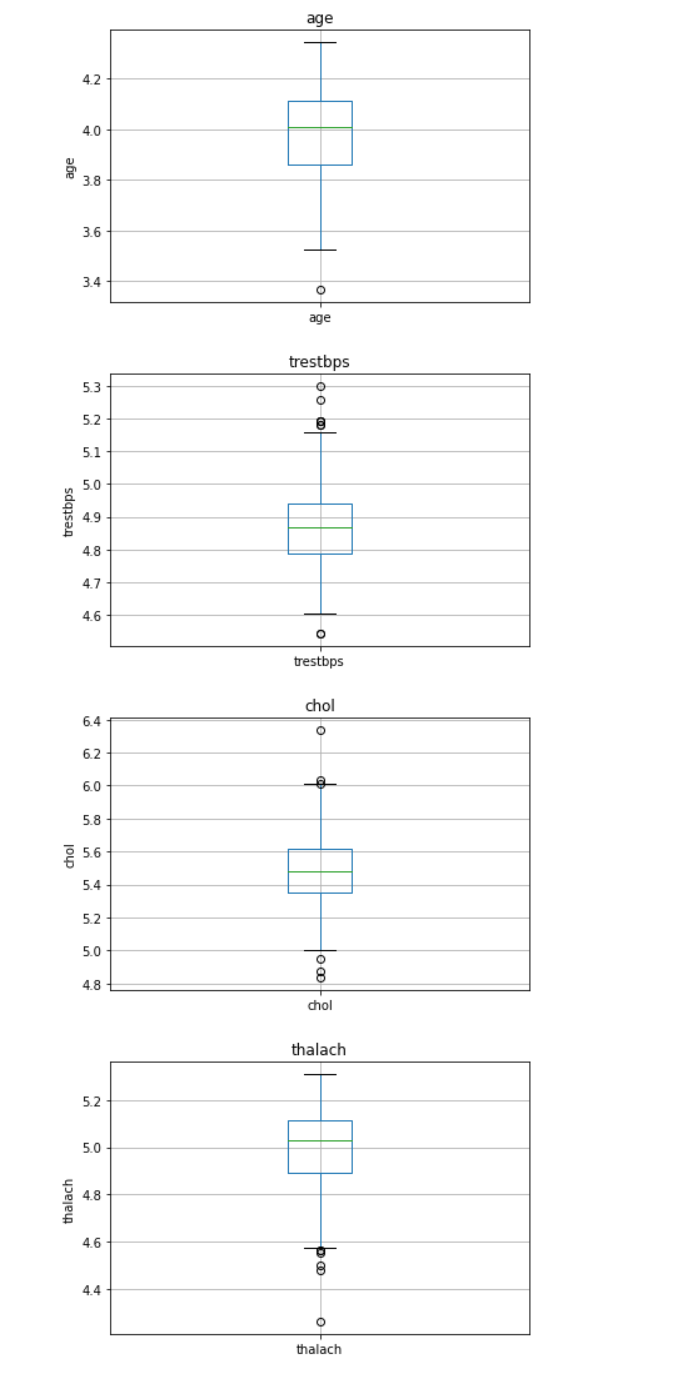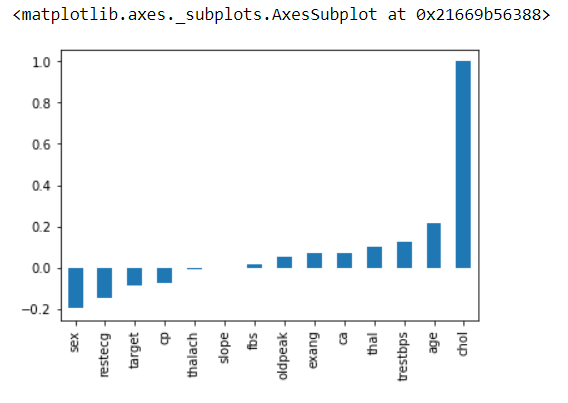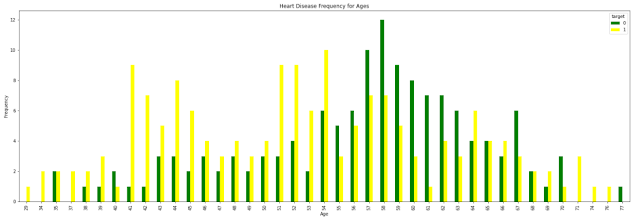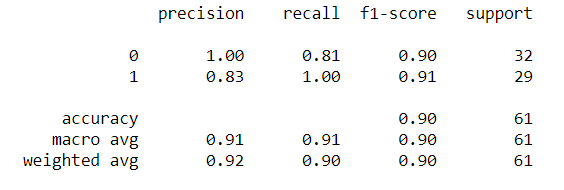## InfinityCodeX

Check out our blogs where we cover topics such as Python, Data Science, Machine Learning, Deep Learning. A Best place to start your AI career for beginner, intermediate peoples.

# (Model Creation)

## Part1: Creating a Machine Learning Model

In this part we are going to write a code to create a machine-learning model which will help us to predict is that person is suffering from heart disease or not.

Data Set For Heart Disease Prediction Project: DATASET

Requirements:  Python >= 3.6

Jupyter Notebook

It's a clean, easy to understand set of data. However, the meaning of some of the column headers is not obvious. Here's what they mean,

1.age: The person's age in years

2.sex: The person's sex (1 = male, 0 = female)

3.cp: The chest pain experienced (Value 1: typical angina, Value 2: atypical angina, Value 3: non-anginal pain, Value 4: asymptomatic)

4.trestbps: The person's resting blood pressure (mm Hg on admission to the hospital)

5.chol: The person's cholesterol measurement in mg/dl

6.fbs: The person's fasting blood sugar (> 120 mg/dl, 1 = true; 0 = false)

7.restecg: Resting electrocardiographic measurement (0 = normal, 1 = having ST-T wave abnormality, 2 = showing probable or definite left ventricular hypertrophy by Estes' criteria)

8.thalach: The person's maximum heart rate achieved

9.exang: Exercise-induced angina (1 = yes; 0 = no)

10.oldpeak: ST depression induced by exercise relative to rest ('ST' relates to positions on the ECG plot. See more here)

11.slope: the slope of the peak exercise ST segment (Value 1: upsloping, Value 2: flat, Value 3: downsloping)

12.ca: The number of major vessels (0-3)

13.thal: A blood disorder called thalassemia (3 = normal; 6 = fixed defect; 7 = reversable defect)

14.target: Heart disease (0 = no, 1 = yes)

### Code:

# Importing Essential Libraries

import pandas as pd
import numpy as np
import matplotlib.pyplot as plt
import seaborn as sns
%matplotlib inline

# calling our CSV file (Your Dataset should be of CSV format)
# To create your CSV file go to Excel file and save as CSV : Ctrl + S } Format==>CSV# Gives Complete information of the data values such as Number of Data present, Null or Not-Null, Data type

data.info()# Counting the target values

data.target.value_counts()

1  165
0  138
Name: target, dtype: int64

# Give a complete statistical description of the Data

data.describe().T# This will show us people who suffer from heart disease with respect to the sex i.e Male and Female

pd.crosstab(data.sex, data.target).plot(kind="bar",figsize=(10,5),color=['blue','red' ])

plt.xlabel('Sex (0 = Female, 1 = Male)') # X-Label

plt.xticks(rotation=0) # Get or set the current tick locations and labels of the x-axis.

plt.legend(["Haven't Disease", "Have Disease"]) # legend = Index

plt.ylabel('Frequency') # X-Label

plt.show() # Help to show our diagram# Finding the numbers of outliers which are present in each feature

Q1 = data.quantile(0.25)
Q3 = data.quantile(0.75)

IQR = Q3 - Q1

((data < (Q1 - 1.5 * IQR)) | (data < (Q3 - 1.5 * IQR))).sum()# Let's Find out the Distribution of Continuous values

for feature in data:
dataset = data.copy()
dataset[feature].hist(bins=25)
plt.xlabel(feature)
plt.ylabel("Count")
plt.title(feature)
plt.show()# Analyzing and treating outliers with a box plot

for feature in data:
dataset = data.copy()

if 0 in dataset[feature].unique():
pass
else:
dataset[feature] = np.log(dataset[feature])
dataset.boxplot(column=feature)
plt.ylabel(feature)
plt.title(feature)
plt.show()# Detecting outliers using z_score
#  z = (x-μ)/σ

outliers = []
def detect_outliers(values):
Threshold = 3
mean_val = np.mean(values)
std_val = np.std(values)

for i in values:
z_score = (i-mean_val)/std_val
if np.abs(z_score) > Threshold:
outliers.append(i)
return outliers

out = detect_outliers(data['age'])
out

[]

outliers = []
def detect_outliers(values):
Threshold = 3
mean_val = np.mean(values)
std_val = np.std(values)

for i in values:
z_score = (i-mean_val)/std_val
if np.abs(z_score) > Threshold:
outliers.append(i)
return outliers

out = detect_outliers(data['trestbps'])
out

[200,192]

outliers = []
def detect_outliers(values):
Threshold = 3
mean_val = np.mean(values)
std_val = np.std(values)

for i in values:
z_score = (i-mean_val)/std_val
if np.abs(z_score) > Threshold:
outliers.append(i)
return outliers

out = detect_outliers(data['chol'])
out

[417, 564, 407, 409]

outliers = []
def detect_outliers(values):
Threshold = 3
mean_val = np.mean(values)
std_val = np.std(values)

for i in values:
z_score = (i-mean_val)/std_val
if np.abs(z_score) > Threshold:
outliers.append(i)
return outliers

out = detect_outliers(data['thalach'])
out



# From the above analysis we can say that we lack the domain knowledge, so it would be best if we just keep all the dataset as it is.

# Finding the correlation between variables

data.corr()['chol'].sort_values().plot(kind='bar')# This will give the correlation between that features

# Example: age in Y-axis and age in X-axis will have the maximum correlation that's why it's dark in color

# annot: Help to see the value inbox

# cmap: The mapping from data values to color space.

# fmt: String formatting code to use when adding annotations.

plt.figure(figsize=(14,10)) # Help to set figure size

sns.heatmap(data.corr(),annot=True,cmap='hsv',fmt='.3f',linewidths=2)

plt.ylim(15,0) # show us the exact number of values we want

plt.show()# This will show us Heart Disease Frequency for Ages

# Target: YES/NO

# color: Green / Yellow

pd.crosstab(data.age,data.target).plot(kind="bar",figsize=(25,8),color=['green','yellow' ])
plt.title('Heart Disease Frequency for Ages')
plt.xlabel('Age')
plt.ylabel('Frequency')
plt.show()# The train_test_split function is for splitting a single dataset for two different purposes: training and testing. The testing subset is for building your model. The testing subset is for using the model on unknown data to evaluate the performance of the model.

# train_test_split is a function in Sklearn model selection for splitting data arrays into two subsets: for training data and for testing data. With this function, you don't need to divide the dataset manually. By default, Sklearn train_test_split will make random partitions for the two subsets. However, you can also specify a random state for the operation.

# .values: This returns back the numpy array

# X, y: The first parameter is the dataset you're selecting to use.

# train_size: This parameter sets the size of the training dataset. There are three options: None, which is the default, Int, which requires the exact number of samples, and float, which ranges from 0.1 to 1.0.

# test_size: This parameter specifies the size of the testing dataset. The default state suits the training size. It will be set to 0.25 if the training size is set to default.

# random_state: The default mode performs a random split using np.random. Alternatively, you can add an integer using an exact number.

from sklearn.model_selection import train_test_split

X = data.drop('target', axis=1).values
y = data['target'].values
X_train, X_test, y_train, y_test = train_test_split(X, y, test_size=0.2, random_state=2)

# Database normalization is the process of structuring a relational database in accordance with a series of so-called normal forms in order to reduce data redundancy and improve data integrity.

# Transform features by scaling each feature to a given range.

# MinMaxScaler: This estimator scales and translates each feature individually such that it is in the given range on the training set, e.g. between zero and one.

# By fit the imputer calculates the means of columns from some data, and by transforming it applies those means to some data (which is just replacing missing values with the means). If both these data are the same (i.e. the data for calculating the means and the data that means are applied to) you can use fit_transform which is basically a fit followed by a transform.

from sklearn.preprocessing import MinMaxScaler

scaler = MinMaxScaler()

X_train = scaler.fit_transform(X_train)
X_test = scaler.transform(X_test)

Creating Machine Learning Model.

### 1)Logistic Regression:

If you want to know more about Logistic Regression, you can definitely check out these blogs which are written in very simple language.

Logistic Regression Blogs:

7 most frequently asked Logistic Regression Questions Answered in 1 blog.

How To Find Optimal Threshold Value And Change Threshold Value In Logistic Regression?

from sklearn.linear_model import LogisticRegression

lr_model = LogisticRegression()

lr_model.fit(X_train,y_train)

lr_pred = lr_model.predict(X_test)

from sklearn.metrics import classification_report, confusion_matrix

print(classification_report(y_test,lr_pred))print(confusion_matrix(y_test,lr_pred))

[[25 7]
[ 1 28]]

### 2)Support Vector Machine:

If you want to know more about Support Vector Machine, you can definitely check out our blog which is written in very simple language.

Support Vector Machine Blog:

Support Vector Machine in Machine Learning

from sklearn import svm

clf_model = svm.SVC(kernel='rbf')

clf_model.fit(X_train,y_train)

clf_pred = clf_model.predict(X_test)

from sklearn.metrics import classification_report, confusion_matrix

print(classification_report(y_test,clf_pred))print(confusion_matrix(y_test,clf_pred))

[[26 6]
[ 1 28]]

### 3)Random Forest:

If you want to know more about Random Forest, you can definitely check out our blog which is written in very simple language.

Random Forest Blog:

Top 10 Strategies Which Will Make You King Of RANDOM FOREST 

from sklearn.ensemble import RandomForestClassifier

rf_model = RandomForestClassifier(n_estimators=200)

rf_model.fit(X_train,y_train)

rf_pred = rf_model.predict(X_test)

from sklearn.metrics import classification_report, confusion_matrix

print(classification_report(y_test,rf_pred))print(confusion_matrix(y_test,rf_pred))

[[26 6]
[ 0 29]]

# RANDOM FOREST GAVE US THE BEST RESULT

import joblib
joblib.dump(rf_model,"Heart_Disease_Prediction.pkl")

['Heart_Disease_Prediction.pkl']

# Testing our model performance
m.predict([[55,1,3,145,233,0,0,150,0,2.2,0,0,1]])

array(, dtype=int64)

So From these, we can conclude that our model is performing great and we are ready to deploy it with the help of flask, which we will see at our next blog.

### Click this link for part 2:

So we hope that you enjoyed this project. If you did then please share it with your friends and spread this knowledge.

Follow us at :

Instagram :

https://www.instagram.com/infinitycode_x/

Heart Disease Prediction End to End Machine Learning Project (Model Creation)Reviewed by InfinityCodeX on Tuesday, October 13, 2020 Rating: 5